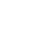Live chatTable of Contents

Data on Visits and Expenditure by UK residents abroad’ from2005Q1 to 2011Q4 was used. It was formatted (appendix 1), and a scatter drawn (appendix 2). The correlation coefficient was calculated and an equation of regression generated. The coefficient of determination was retrieved from the equation. The following was then obtained from the appendices.

The source of the data and the reasons why the variables might be related.

This data was obtained from the Office of National Statistics (ONS) and was downloaded from their website http://www.ons.gov.uk.  The variables in this data are related. The total amount of expenditure that UK residents use depends on how many people made the visits and how many times those who made these visits make them. In this case, the amount of expenditure will depend on the number of visits made. If no visits are made, there will be no expenditure.

An interpretation of the value of the correlation coefficient in the context of the data.

The correlation coefficient, denoted as r, shows how closely related or not related variables are. When r is a negative number, the variables are negatively related and an increase in one leads to a decrease in the other. Positive r value shows that an increase of one variable leads to the increase of the other. In our data, y-axis represents the number of visits while x-axis represents the amount of expenditure. The r-value is 0.8340807. This shows that the two variables are highly correlated and an increase in visits leads to an increase in expenditure.

State the equation of the regression line in the context of the meaning of the data (write the equation of the regression line)

The equation is y=2.444x-4476. It means that any value of y, which is the expenditure, is two times the number of visits, represented by x, less 4476 million pounds

## An interpretation of the intercept and the gradient

The gradient of a graph shows the strength of the trend between the two variables being investigated. A steep gradient shows a strong trend while a slightly slant gradient shows a weak trend. A negative gradient represents a decreasing trend. In our data, the equation y=2.444x-4476 shows that the gradient is 2.444 which is positive. It shows that the number of visits at any given point is 2.444 times the expenditure, less 4476, which is the intercept. We can therefore, from this, be able to estimate the number of visits that we need in order to obtain a certain target in expenditure and vice versa. The negative intercept means that if there is no expenditure, the number of visits is negative, which could mean that there are foreign visitors coming into the UK.

An interpretation of the value of the coefficient of determination (R2)

R2 is used to test the goodness of fit of the model. The higher the value, the better the model and the more reliable it can b used to predict the future. An R2 value of 1 shows a perfect model while a value of zero shows that the data cannot be adequately used to forecast the outcome. Experts advise that an R2 value of 0.39 or less should not be used in statistical analysis since it is unreliable. In our data, R2 value is 0.6956906 which gives the data and graph credibility. It can be used for future forecasts or planning purposes in the tourism department in the UK.

Show how your regression equation can be used to make predictions. Comment on the accuracy of these predictions. (Use the regression equation and predict two values then comment how accurate the values are).

Stay ConnectedThe regression equation can be used to make predictions. This is because R2 is high enough to be reliable at 0.6956906. To predict the future, the government can use equation by putting a ceiling on the amount that they wish to spend in foreign visits. This will help determine the number of visits needed to obtain that figure. For instance, if the government wishes to have a total expenditure of 10000 million, the equation will be applied as

y=2.444x10000)-4476

y therefore = 19964000visits

If there are 15 million expected foreign visits, y=15000 and x can be calculated as

15000=2.444x-4476

X= (15000+4476)/2.444

X=7968.9 million pounds

 y=2.4443x-4476.9 UK visits abroad: expenditure £million=2.4443*UK visits abroad: all visits -4476.9 enterpolation UK visits abroad: expenditure £million = 2.4443*16957-4476.9= 36971.1 extrapolation UK visits abroad: expenditure £million = 2.4443*12000-4476.9= 24854.7

0

Preparing Orders

0

Active Writers

0

Support Agents

Limited offerget 15% off your 1st order with code first15